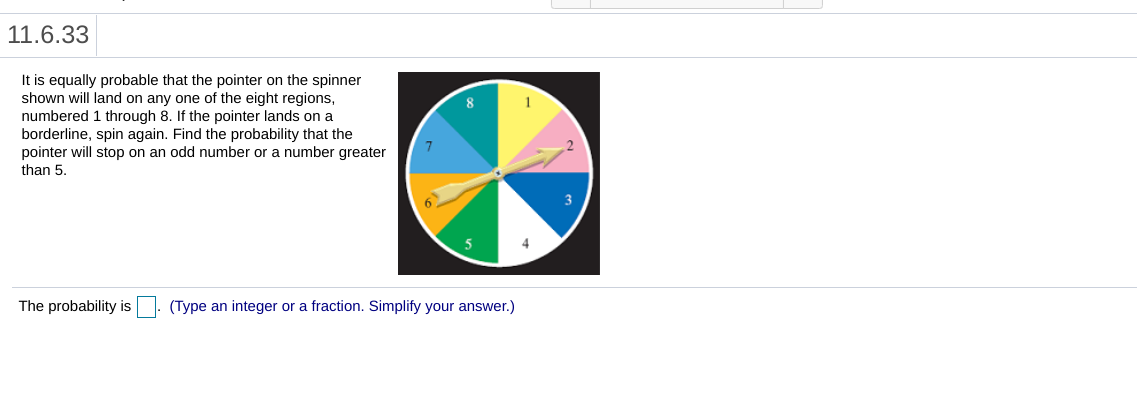# 11.6.33It is equally probable that the pointer on the spinnershown will land on any one of the eight regionsnumbered 1 through 8. If the pointer lands on aborderline, spin again. Find the probability that thepointer will stop on an odd number or a number greaterthan 5The probability isType an integer or a fraction. Simplify your answer.)

Questionhelp_outlineImage Transcriptionclose11.6.33 It is equally probable that the pointer on the spinner shown will land on any one of the eight regions numbered 1 through 8. If the pointer lands on a borderline, spin again. Find the probability that the pointer will stop on an odd number or a number greater than 5 The probability is Type an integer or a fraction. Simplify your answer.) fullscreen
check_circleExpert Solution
Step 1

It is possible for the pointer to land on a number that is both Odd and greater than 5.

number  Odd numbers {1,3,5,7} is 4,

Number Greater than 5  {6,7,8} is 3

7 is only number which  Odd and greater than 5.

The probability of landing on a number that is Odd or greater than 5 is calculated as follows:

Step 2

These events are not mut...

### Want to see the full answer?

See Solution

#### Want to see this answer and more?

Solutions are written by subject experts who are available 24/7. Questions are typically answered within 1 hour*

See Solution
*Response times may vary by subject and question
Tagged in

### Basic Probability